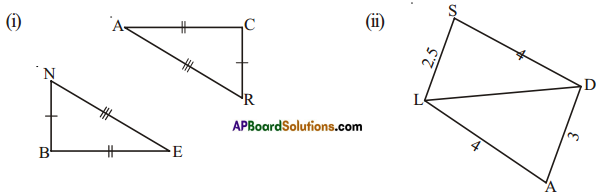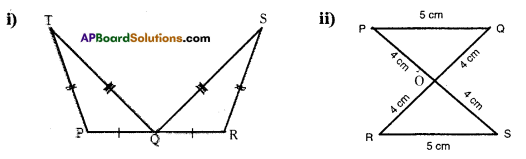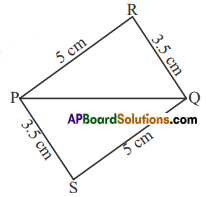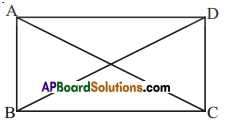# HSSlive: Plus One & Plus Two Notes & Solutions for Kerala State Board

## AP Board Class 7 Maths Chapter 8 Congruency of Triangles Ex 1 Textbook Solutions PDF: Download Andhra Pradesh Board STD 7th Maths Chapter 8 Congruency of Triangles Ex 1 Book AnswersAP Board Class 7 Maths Chapter 8 Congruency of Triangles Ex 1 Textbook Solutions PDF: Download Andhra Pradesh Board STD 7th Maths Chapter 8 Congruency of Triangles Ex 1 Book Answers

## Andhra Pradesh State Board Class 7th Maths Chapter 8 Congruency of Triangles Ex 1 Books Solutions

 Board AP Board Materials Textbook Solutions/Guide Format DOC/PDF Class 7th Subject Maths Chapters Maths Chapter 8 Congruency of Triangles Ex 1 Provider Hsslive

2. Click on the Andhra Pradesh Board Class 7th Maths Chapter 8 Congruency of Triangles Ex 1 Answers.
3. Look for your Andhra Pradesh Board STD 7th Maths Chapter 8 Congruency of Triangles Ex 1 Textbooks PDF.
4. Now download or read the Andhra Pradesh Board Class 7th Maths Chapter 8 Congruency of Triangles Ex 1 Textbook Solutions for PDF Free.

## AP Board Class 7th Maths Chapter 8 Congruency of Triangles Ex 1 Textbooks Solutions with Answer PDF Download

Find below the list of all AP Board Class 7th Maths Chapter 8 Congruency of Triangles Ex 1 Textbook Solutions for PDF’s for you to download and prepare for the upcoming exams:

Question 1.
Decide whether the SSS congruence is true with the following figures. Give reasonsSolution:
i) From the figures,
BE = CA
EN = AR
BN = CR
∴ S.S.S congruency is true.

ii) From the figure,
AL = SD = 4 cm
LD = LD = common side
AD ≠ LS (3 cm ≠ 2.5 cm)
∴ S.S.S congruency is not ture.

Question 2.
For the following congruent triangles, and the pairs of corresponding angles.Solution:
i) ∠P = ∠R
∠T = ∠S
∠TQP = ∠SQR

ii) ∠P=∠S
∠Q = ∠R
∠POQ = ∠SOR

Question 3.
(i) ΔPQR ≅ ΔPQS
(ii) ΔPQR ≅ ΔQPS
(iii) ΔPQR ≅ ΔSQP
(iv) ΔPQR ≅ ΔSPQSolution:
(ii) ΔPQR ≅ ΔQPS is correct.

Question 4.
In the figure given below, AB = DC and AC = DB. Is ΔABC ≅ ΔDCB.Solution:
Given that AB = DC
AC = DB
Also from the figure ∠B = ∠C
∴ ΔABC ≅ ΔDCB

## Andhra Pradesh Board Class 7th Maths Chapter 8 Congruency of Triangles Ex 1 Textbooks for Exam Preparations

Andhra Pradesh Board Class 7th Maths Chapter 8 Congruency of Triangles Ex 1 Textbook Solutions can be of great help in your Andhra Pradesh Board Class 7th Maths Chapter 8 Congruency of Triangles Ex 1 exam preparation. The AP Board STD 7th Maths Chapter 8 Congruency of Triangles Ex 1 Textbooks study material, used with the English medium textbooks, can help you complete the entire Class 7th Maths Chapter 8 Congruency of Triangles Ex 1 Books State Board syllabus with maximum efficiency.

## FAQs Regarding Andhra Pradesh Board Class 7th Maths Chapter 8 Congruency of Triangles Ex 1 Textbook Solutions

#### Can we get a Andhra Pradesh State Board Book PDF for all Classes?

Yes you can get Andhra Pradesh Board Text Book PDF for all classes using the links provided in the above article.

## Important Terms

Andhra Pradesh Board Class 7th Maths Chapter 8 Congruency of Triangles Ex 1, AP Board Class 7th Maths Chapter 8 Congruency of Triangles Ex 1 Textbooks, Andhra Pradesh State Board Class 7th Maths Chapter 8 Congruency of Triangles Ex 1, Andhra Pradesh State Board Class 7th Maths Chapter 8 Congruency of Triangles Ex 1 Textbook solutions, AP Board Class 7th Maths Chapter 8 Congruency of Triangles Ex 1 Textbooks Solutions, Andhra Pradesh Board STD 7th Maths Chapter 8 Congruency of Triangles Ex 1, AP Board STD 7th Maths Chapter 8 Congruency of Triangles Ex 1 Textbooks, Andhra Pradesh State Board STD 7th Maths Chapter 8 Congruency of Triangles Ex 1, Andhra Pradesh State Board STD 7th Maths Chapter 8 Congruency of Triangles Ex 1 Textbook solutions, AP Board STD 7th Maths Chapter 8 Congruency of Triangles Ex 1 Textbooks Solutions,
Share: